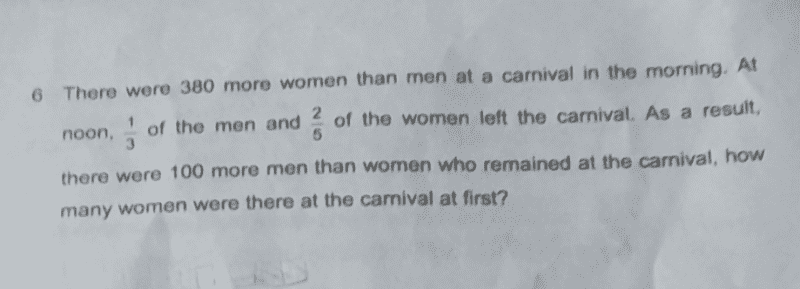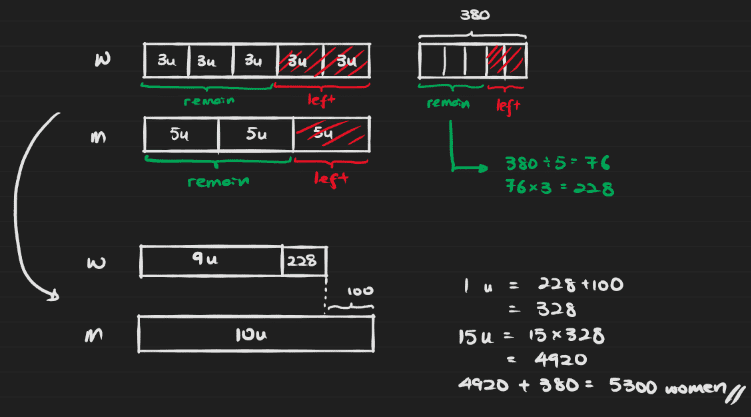# QuestionHi all..how do I solve using model method?

Is there a website to learn these fractions methods? I’m trying to learn..This is a specific model drawing technique called model stacking in some schools. You can refer to the YouTube video link to learn more. It basically uses models to solve questions that would otherwise require simultaneous equations.

0 Replies 2 Likes ✔Accepted Answer

Er… I don’t know how to solve using models.

There are plenty of books around.  I was looking through some PSLE books in Popular bookstore and they have quite a number from \$8 – \$20.  Can buy 🙂

For the problem, let me solve by algebra.  I’ll let someone else follow up with the model method.

Let M = Number of men at the carnival originally
Let W = Number of women at the carnival originally

(1) M = W – 380

(2) 2/3 M – 3/5W = 100
=> M – (3/2)(3/5)W = (3/2)100
=> M – (9/10)W = 150

Substituting (1) into (2), we get
W – 380 – (9/10)W = 150
=> (1/10)W = 380 + 150 =  530
=> W = 530 * 10 = 5300 #

0 Replies 2 Likes